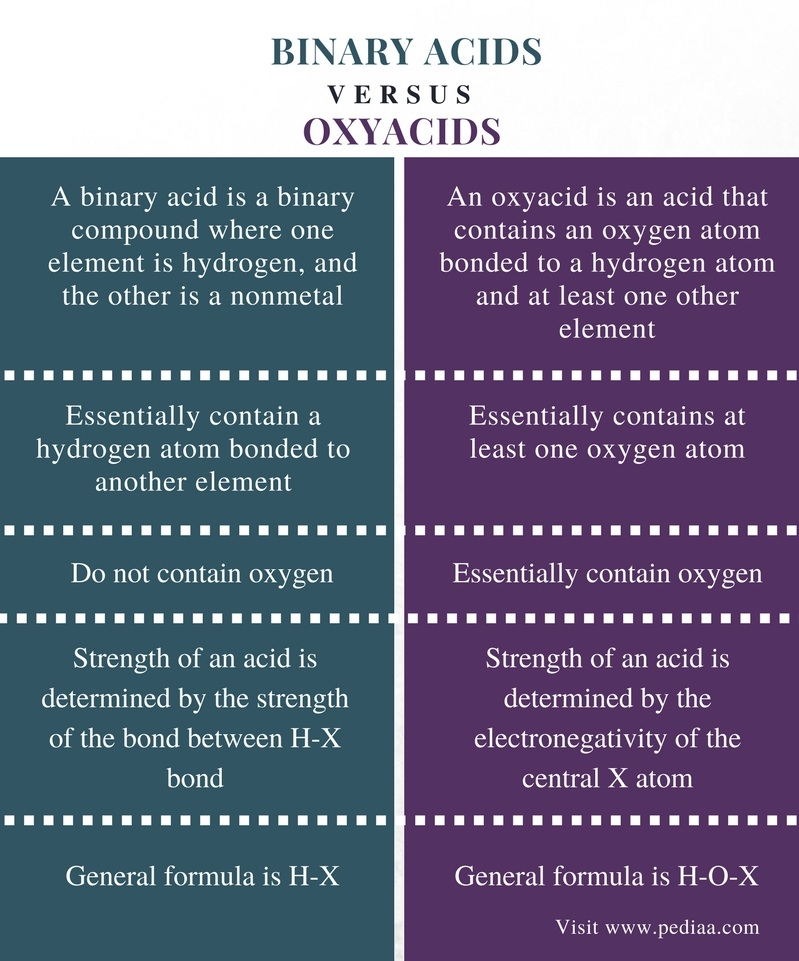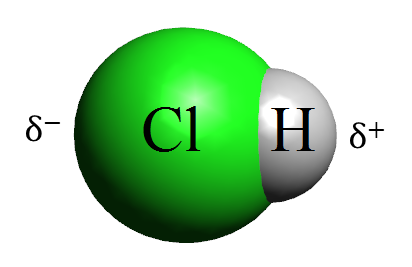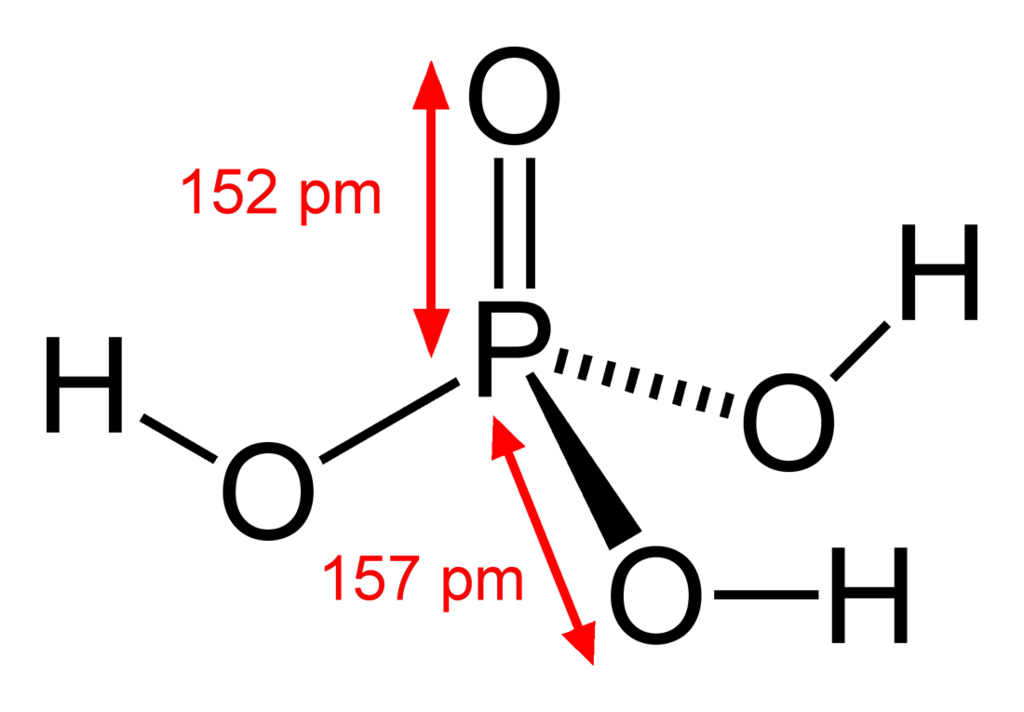# Distinguish Between Binary Acids and Oxyacids

## Main Difference – Binary Acids vs Oxyacids

Binary acids and oxyacids are two types of acidic compounds. Binary acids are compounds that always contain a hydrogen atom bonded to a different element; hence they are also known as hydracids. Here, the hydrogen atom is bonded to a nonmetal such as a halogen, sulfur, etc. Oxyacids are compounds that essentially contain oxygen. The main difference between binary acids and oxyacids is that binary acids have the general formula H-X whereas oxyacids have the general formula H-O-X. Thus, the general formula of these acids helps to distinguish between binary acids and oxyacids.

### Key Areas Covered

1. What are Binary Acids
– Definition, Examples
2. What are Oxyacids
– Definition, Properties, Examples
3. How to Distinguish Between Binary Acids and Oxyacids
– Comparison of Key Differences

Key Terms: Acid, Binary Acid, Electronegativity, Halogen, Hydracids, Oxyacid, Nonmetal## What are Binary Acids

A binary acid is a binary compound where one element is hydrogen, and the other is a nonmetal. These compounds are also known as hydracids because they essentially have hydrogen. The nonmetal is a chemical element in the p block of the periodic table of elements. Binary acids are not always diatomic molecules; they just have two different elements bonded to each other. The general formal is H-X.Figure 1: HCl is a Binary Acid

Binary acids are capable of donating hydrogen atoms to the medium (H+). The nomenclature of binary acids has the same structure. If the binary acid is in the pure form, the name starts with “hydrogen”, and the anionic name ends with “-ide”. Followings are some examples of binary acids.

• Diatomic binary acids – HCl, HI, etc.
• Polyatomic binary acids – H2S
• Halogen-containing binary acids – HF, HCl, HBr and HI

## What are Oxyacids

An oxyacid is an acid that contains an oxygen atom bonded to a hydrogen atom and at least one other element. The general structure of an oxyacid is H-O-X. A compound having this formula can dissociate in the aqueous medium in two different ways as given below.

X−O−H    ⇄     (X−O)−   +   H+

X−O−H   ⇄       X+      +    OH

If the X atom has a high electronegativity, then the electrons of oxygen atom are attracted by it. Then the bond between oxygen and hydrogen becomes weak. As a result, the hydrogen atom is released as given in the first equation. This type of reaction is given by oxyacids.Figure 2: Phosphoric Acid is an Oxyacid

But if the electronegativity of X is low, it tends to release hydroxide ions as given in the second equation. Ex: NaOH. These compounds can be sometimes amphoteric if the electronegativity of X is a moderate value. This type of reactions is given by “oxoacids”.

### Examples:

• Oxyacids of sulfur – H2SO4, H2SO3
• Oxyacids of phosphorous – H3PO4
• Oxyacids of nitrogen – HNO3, HNO2

## Distinguish Between Binary Acids and Oxyacids

### Definition

Binary Acids: A binary acid is a binary compound where one element is hydrogen, and the other is a nonmetal.

Oxyacids: An oxyacid is an acid that contains an oxygen atom bonded to a hydrogen atom and at least one other element.

### Components

Binary Acids: Binary acids essentially contain a hydrogen atom bonded to another element.

Oxyacids: Oxyacids essentially contains at least one oxygen atom.

### Oxygen

Binary Acids: Binary acids do not contain oxygen.

Oxyacids: Oxyacids essentially contain oxygen.

### Strength

Binary Acids: The strength of an acid is determined by the strength of the bond between H-X bond in binary acids.

Oxyacids: The strength of an acid is determined by the electronegativity of the central X atom in oxyacids.

### General Formula

Binary Acids: The general formula of binary acids is H-X.

Oxyacids: The general formula of oxyacids is H-O-X.

### Conclusion

Binary acids are compounds containing hydrogen atoms bonded to a different chemical element. Oxyacids are acidic compounds containing at least one oxygen atom. It is the general formula of these acids that helps to distinguish between binary acids and oxyacids; binary acids have the general formula H-X whereas oxyacids have the general formula H-O-X.

##### Reference:

1. Helmenstine, Anne Marie. “Definition of Binary Acid.” ThoughtCo, Jun. 23, 2014, Available here.
2. Helmenstine, Anne Marie. “Oxyacid Definition and Examples.” ThoughtCo, Oct. 10, 2017, Available here.
3. Zumdahl, Steven S. “Oxyacid.” Encyclopædia Britannica, Encyclopædia Britannica, inc., 15 Aug. 2008, Available here.

##### Image Courtesy:

1. “Dipolna molekula HCl” By Drago Karlo – Own work (CC BY-SA 4.0) via Commons Wikimedia
2. “Phosphoric-acid-2D-dimensions” (Public Domain) via Commons Wikimedia